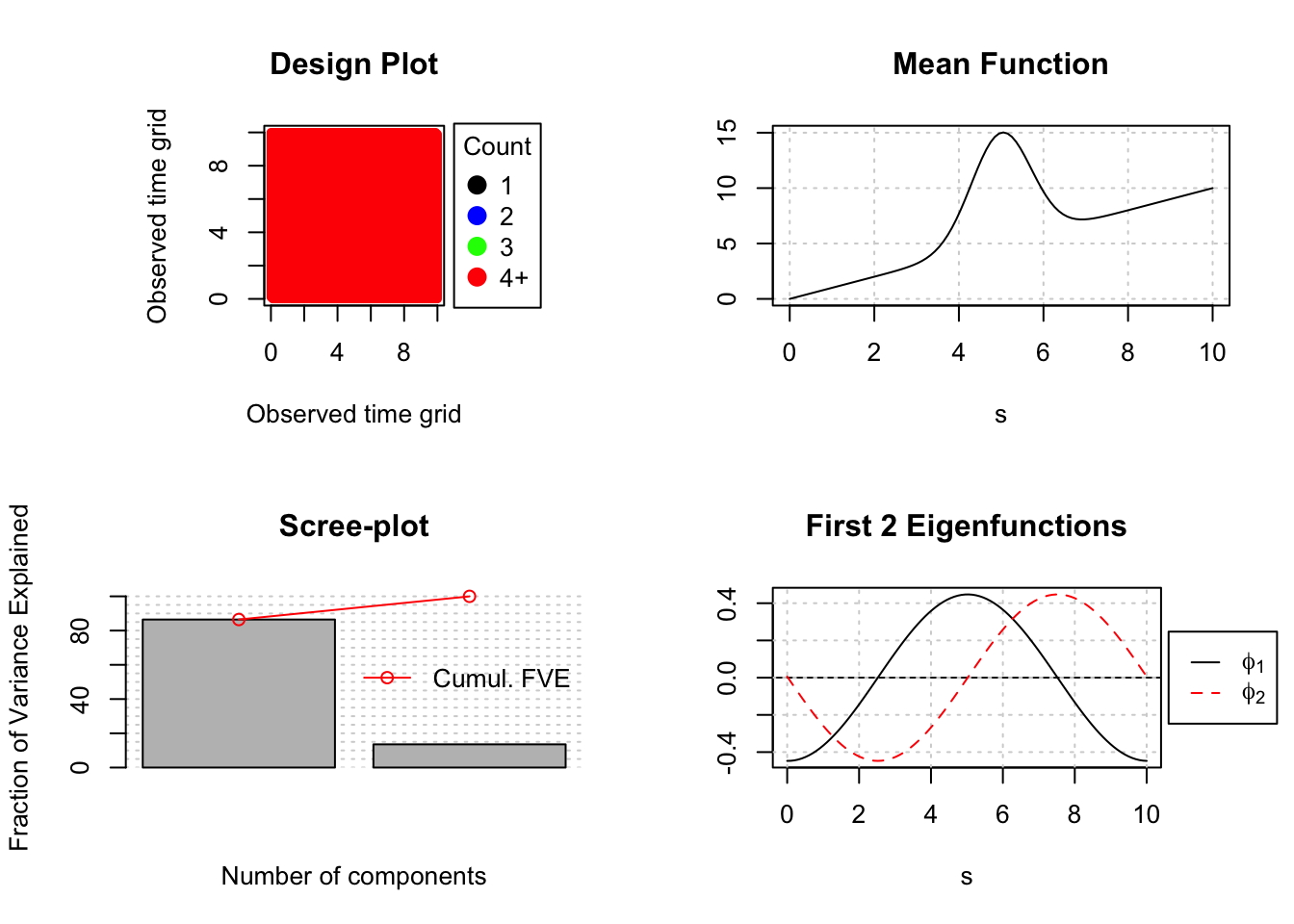## Overview

The basic work-flow behind the PACE approach for sparse functional data is as follows (see eg. (Yao, Müller, and Wang 2005; Liu and Müller 2009) for more information):

1. Calculate the smoothed mean $$\hat{\mu}$$ (using local linear smoothing) aggregating all the available readings together.
2. Calculate for each curve separately its own raw covariance and then aggregate all these raw covariances to generate the sample raw covariance.
3. Use the off-diagonal elements of the sample raw covariance to estimate the smooth covariance.
4. Perform eigenanalysis on the smoothed covariance to obtain the estimated eigenfunctions $$\hat{\phi}$$ and eigenvalues $$\hat{\lambda}$$, then project that smoothed covariance on a positive semi-definite surface (Hall, Müller, and Yao 2008).
5. Use Conditional Expectation (PACE step) to estimate the corresponding scores $$\hat{\xi}$$. ie. $$\hat{\xi}_{ik} = \hat{E}[\hat{\xi}_{ik}|Y_i] = \hat{\lambda}_k \hat{\phi}_{ik}^T \Sigma_{Y_i}^{-1}(Y_i-\hat{\mu}_i)$$.

As a working assumption a dataset is treated as sparse if it has on average less than 20, potentially irregularly sampled, measurements per subject. A user can manually change the automatically determined dataType if that is necessary. For densely observed functional data simplified procedures are available to obtain the eigencomponents and associated functional principal components scores (see eg. (Castro, Lawton, and Sylvestre 1986) for more information). In particular in this case we:

1. Calculate the cross-sectional mean $$\hat{\mu}$$.
2. Calculate the cross-sectional covariance surface (which is guaranteed to be positive semi-definite).
3. Perform eigenanalysis on the covariance to estimate the eigenfunctions $$\hat{\phi}$$ and eigenvalues $$\hat{\lambda}$$.
4. Use numerical integration to estimate the corresponding scores $$\hat{\xi}$$. ie. $$\hat{\xi}_{ik} = \int_0^T [ y(t) - \hat{\mu}(t)] \phi_i(t) dt$$

In the case of sparse FPCA the most computational intensive part is the smoothing of the sample’s raw covariance function. For this, we employ a local weighted bilinear smoother.

A sibling MATLAB package for fdapace can be found in here.

## FPCA in R using fdapace

The simplest scenario is that one has two lists yList and tList where yList is a list of vectors, each containing the observed values $$Y_{ij}$$ for the $$i$$th subject and tList is a list of vectors containing corresponding time points. In this case one uses:

FPCAobj <- FPCA(Ly=yList, Lt=tList)

The generated FPCAobj will contain all the basic information regarding the desired FPCA.

### Generating a toy dense functional dataset from scratch

  library(fdapace)

# Set the number of subjects (N) and the
# number of measurements per subjects (M)
N <- 200;
M <- 100;
set.seed(123)

# Define the continuum
s <- seq(0,10,length.out = M)

# Define the mean and 2 eigencomponents
meanFunct <- function(s) s + 10*exp(-(s-5)^2)
eigFunct1 <- function(s) +cos(2*s*pi/10) / sqrt(5)
eigFunct2 <- function(s) -sin(2*s*pi/10) / sqrt(5)

# Create FPC scores
Ksi <- matrix(rnorm(N*2), ncol=2);
Ksi <- apply(Ksi, 2, scale)
Ksi <- Ksi %*% diag(c(5,2))

# Create Y_true
yTrue <- Ksi %*% t(matrix(c(eigFunct1(s),eigFunct2(s)), ncol=2)) + t(matrix(rep(meanFunct(s),N), nrow=M))

### Running FPCA on a dense dataset

  L3 <- MakeFPCAInputs(IDs = rep(1:N, each=M), tVec=rep(s,N), t(yTrue))
FPCAdense <- FPCA(L3$Ly, L3$Lt)

# Plot the FPCA object
plot(FPCAdense)# Find the standard deviation associated with each component